## The Dirac equation

### The real thing

1. Charged spin-½ particles: Two spinors
2. Linearisation
3. Equation of motion for particle and antiparticle
4. Relativistic invariance: The Dirac algebra

CONNECTION TO KLEIN-GORDON EQUATION

Multiplying the second equation from the left with the operator of the first (or vice versa) yields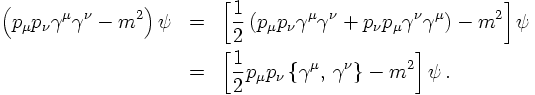The resulting operator becomes the kernel of the Klein-Gordon equation only, if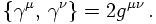This infact defines the γ matrices. An explicit representation in terms of the Pauli matrices and the unit matrix is given by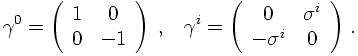Since, after explicit calculation in this representation,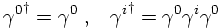the E.o.M. for the daggered spinor can now be obtained from the one for the undaggered one: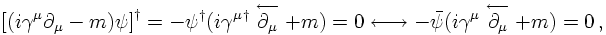as before. The trick here was to multiply from the right with γ0 in the last step.

CURRENT AND CHARGE

Combining the E.o.M. for the barred and the unbarred spinors, i.e. adding them, yields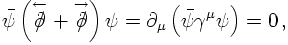a perfect candidate for a conserved current. The corresponding density is positive, by construction, and therefore perfectly suited to describe a particle density. Needless to stress that the current transforms like a four-vector.

STATES

In a next step, let's try to construct plane wave solutions of the Dirac equation with positive or negative energies: Both signs should appear, after all, the Dirac equation is a linearisation of the Klein-Gordon equation involving more degrees of freedom, but it should now be possible to map the different energy signs onto specific solutions. So, for this case, let's try an ansatz of the form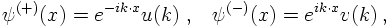where the signs in the superscript indicate the sign of the energy. The Dirac equation now implies that for particles at rest the two solutions must satisfywhere the entries in the matrices are to be understood as 2x2 matrices. This shows that the solutions given above for the eigenstates are indeed deeply rooted in the form of the E.o.M.s.
Starting form these solutions for the particles at rest, it would be simple to perform a boost in order to obtain the solutions for the particles moving. However, rather than so doing, it is simpler to observe that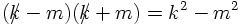and to write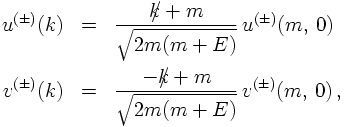where in both cases E is defined as the corresponding positive quantity. Now, it is time to specify the normnalisation factor N in front of the spinors at rest. It will turn out that a convenient choice is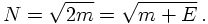The latter identity of course holds true only for particles at rest, where E = m. For the conjugate spinors similar equations hold true, i.e.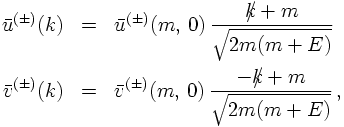implying that the base states are orthogonal with norm N: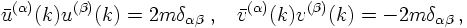with all other combinations vanishing. The quantisation of the Dirac field proceeds in the usual way: For each degree of freedom a corresponding creation operator is introduced; their complex conjugates being the annihilation operators. Therefore, altogether four creation operators are defined, which are multiplied with "their" respective spinor component and a plane wave factor. However, this will not be discussed here in great detail.

PROJECTORS ON POSITIVE OR NEGATIVE ENERGIES

Consider now the matrices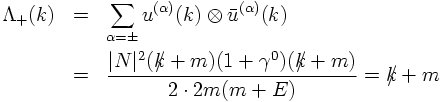and similarly for the negative energy states,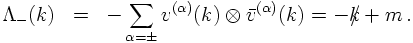They serve as projectors onto positive and negative energy states. Their sum equals 2m, squaring them results - up to a factor of 2m - in the original projector.

5. Spin, helicity and chirality
6. The parity of the Dirac spinors